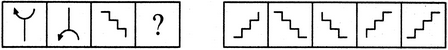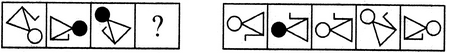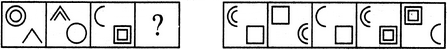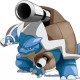Non-Verbal Analogy

Reasoning requires logical and intellectual skills as well as practice. One of the most important parts of this reasoning is the non-verbal analogy. It is important that you know the shortcuts and tricks so that you can solve these questions quickly because time is the most important factor. These tricks can help you solve questions in bank exams, government exams, and many other exams that have a time limit.

Suggested VideosWhat is a Non-verbal Analogy?

When you compare two things it becomes an analogy. And in the non-verbal analogy, you compare two similar figure and find the relationship between them. You can easily master this topic because unlike other questions this one does not require any numerical skill. Also, you don’t need to remember any formula while solving the question.Non-verbal analogy

Now we will help you with some of the solved questions. This will help you understand non-verbal analogy questions better. Later we will provide you with some of the new questions for practice.

Rotation of the Figures

In this type of non-verbal analogy questions, you need to establish a similarity between the first figures and then apply the same rule to the other two figures and find the missing figure. If the question is based on rotation than you need to determine how much do the figures in the question rotates in comparison each other. Once you have established the amount of rotation than you need to check on the other two i.e. one in the question and one out of the figures given in the answer to check which is the correct figure.

Example 1: Which suitable figure will replace the question mark based on the figures given in question?In this question, we need to find the similarity between the first two figures. Looking at these two figures you can see that figure B is rotated 180 to the right of the figure A. The same logic applies to the other two figures. From the options, you can see that when you rotate figure C by 180° you will get the figure given in option 2. Thus the correct answer is option 2.

From the given figures find the suitable figure that can replace the question mark.Figure A and B are vertically inverted to each other. In simple terms when you see the figure A in the mirror invertedly you will see a figure similar to the one given in B. Applying the same logic, the figure in option (1) will be vertically inverted to the figure C. Thus a correct answer is an option (1).

Example 2: Establish the similar relationship between C and D as the one given in figure A and B.Here either side of figure A is not shaded, whereas the left side of figure B is shaded black. Similarly, figure C is not at all shaded and the upper portion of figure D will be shaded. Thus the correct answer here is a figure (5).

Practice Questions

Q1. Which suitable figure will complete the question mark in the below figure.A. 1                       B. 2

C. 3                      D. 4

Ans: C) 3

Q. Based on the figure in the question find a suitable figure from the five figures in the option.A. 1                      B. 2

C. 3                      D. 4

Ans: C) 3

Q. Select a suitable figure that will replace the question mark of the figure given in the question.A. 1                     B. 2

C. 3                    D. 4

E. 5

Share with friends

Customize your course in 30 seconds

Which class are you in?
5th
6th
7th
8th
9th
10th
11th
12th
Get ready for all-new Live Classes!
Now learn Live with India's best teachers. Join courses with the best schedule and enjoy fun and interactive classes.Ashhar Firdausi
IIT Roorkee
BiologyDr. Nazma Shaik
VTU
ChemistryGaurav Tiwari
APJAKTU
Physics
Get Started

Browse

Analogy3 Followers

Most reacted comment
43 Comment authorsRecent comment authors
Subscribe
Notify ofGuest
Sai Rohith

5:62::8:?, Solve the problemGuest

5:62

5:5*12+2

8: 8*12+12

or

5:6+7*8
8:9+10*11

seriously options are key to find the patternGuest
Vicky

5×12=60
Now 60+2=62
Similarly 8×12=96
So,96+2=98Guest
Arya

140Guest
Raju

98Guest
sai sameer

ans: 5 square revers +1, 8 square reverse +1=47Guest
Geetanjali Malik

5:62::8:?
5*12=60+2
=62
In the same way
8*12=96+2
=98Guest
KARAN singh ThakurGuest
Raj

8:98
Here I explain
9+8=17,

8:98.Guest
Sai AshrithaGuest
Keshav Sharma

2:12 :: 3 : solveGuest
Jalaj

18Guest
Ilyas

18Guest
Lucky

68:100::45:?Guest
Tera Gaand Maar Dunga

77Guest
Muskan

81Guest
Aman

6^2 + 8^2= 100
4^2+ 5^2= 41..Guest
Sam

15:50::80:?Guest
Sam

and howGuest
Anonymous

18Guest
Akhalesh Kumar

15*3+5=50
80*3+5=245Guest
Manoj

39:143::221:?Guest
sujatha

1011:10111::11111:
find next number analogyGuest
Akhalesh Kumar

1011*10+1=10111
11111*10=111110+1=111111Guest
marc

8:100::12:? solve this problemGuest
sai kiran reddy

4*2=8
4*25=100
6*2=12
6*25=150Guest
Ravi Sagar

63:30::94:?
a) 54
b) 58
c)112
d) 52Guest
Abdullah umar

if x=144 and y=18 what will be x:y=Guest
Manna

x=144, y=18.
Therefore, x:y = 144:18.
144 and 18 have 18 as highest common factor (highest number which can divide both).
144/18=8. This implies, 144/18:18/18
Therefore, 144: 18= 8:1.Guest
Ankit

7:350 ::. ?
1) 4: 216
2) 5: 625
3) 6 : 222
4) 8: 636Guest
Saif

12:312::10:? SolveGuest
TUSAR RANJAN PANIGRAHI

Ans-220
(10)^2+10(10+2)Guest
Vikas

60:16::100:? Solve kijiyeGuest
Vikas

60:16::100:?Guest
jyoti rawat

10*6=60
10+6=16
10*10=100
10+10=20Guest
Jay

46:22::76:55::81:65::36:15::27:?
Solve thisGuest
Jay

23:29::35:128::52:13:94:?Guest
RAM KRISHNA

26:39 the analogy is 19:494 how? Prove me sirGuest
Maaz

GO-484, BY-927, HE-?Guest
Pragya kumar

961Guest
guest

Q 1: If x = 144 and y = 18, then x : y what

96: 12
13: 169
169: 13
256: 16Guest
Pragya kumar

96:12Guest
guest

Q 2: Guess the next number: 19 : 361 :: 66 : ____

693
256
4356
5346Guest
Pragya kumar

4356Guest
Smruti Ranjan Mohapatra

fake site don’t do anything, don’t completly rely on this site , no reply , no answer, only fake assurance, no attendant present, no responsibility, the only thing I know about them is that they want only money for their business nothing else.Guest
Sohanpal Kashyap

34:21: ::124:? Help any oneGuest
Amarendraparida

14:20::81:?Guest
Prince

Slove 19:399::21?Guest
Ekdkk

483Guest
Babli Kumari

128 147 164 173 wrong number find with explainsGuest
Shree

find analog 7:13::9:?Guest
sunil

2:12::3:? solve the questionGuest

PST:01::NPR:?, Solve the problemGuest
Gowda

228:56::336: ?

1.108
2.63
3.72
4.36Guest
Shivi manhas

18:5::12:? Solve the problmGuest
Arun Gyanba KambleGuest
aish

14Guest
Syed shamsi

Solve 123 : 216 :: 245 😕Guest
Sneha

55:25 :: 73:?Guest

48:122::168:?Guest

PEN=16 BOAY=6

PAMPI=?

1. 21

2. 23

3. 20

4. 19Guest
Guest

8: 72::12: ? Solve the problemGuest
S.D.R

29:59::76:?
solve the problemGuest
Arup bhattacharya

72:81::64:?